Home > A2C > Chapter 9 > Lesson 9.3.2 > Problem9-147

9-147.
1. Sketch the graph of each equation below. Homework Help ✎

1.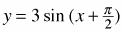2. y = −2 sin (4x)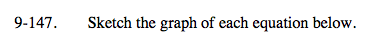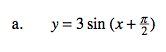$\text{locator }\left(-\frac{\pi}{2}, 0\right)$

period = 2π

amplitude = 3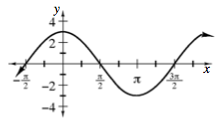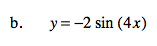locator (0, 0)

$\text{period=}\frac{\pi}{2}$

amplitude = 2

inverted